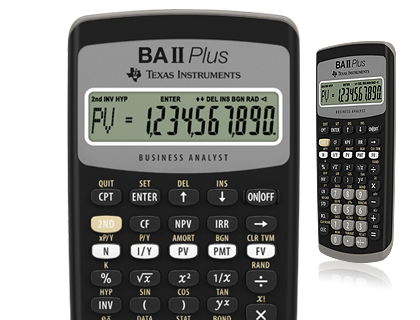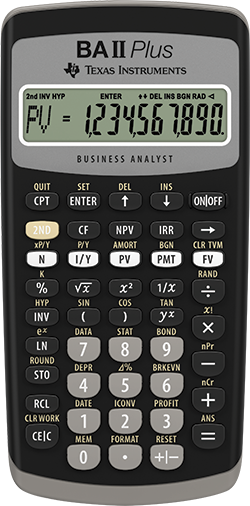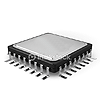# BA II Plus™ financial calculator

Designed for business professionals and students, this easy-to-use financial calculator delivers powerful computation functions and memory.#### Key features

• Time-Value-of-Money (TVM) calculations
• Amortization schedules
• Cash-flow analysis, Net Present Value (NPV) and Internal Rate of Return (IRR)
• Depreciation with four different methodologies
• Breakeven, profit and percent difference calculations
• Second key feature to calculate terms fast

## Exam acceptance

The BA II Plus™ is approved for use on the following professional exams:
• Chartered Financial Analyst®* exam
• GARP® Financial Risk Manager (FRM®)** exam
• Certified Management Accountants®*** exam

## Feature highlights

Prompted display shows current variable label and values.
• Calculate IRR and NPV for cash-flow analysis
Store up to 24 uneven cash flows with up to four-digit frequencies; edit inputs to analyze the impact of changes in variables.
• Time-value-of-money function
Quickly solve calculations for annuities, loans, mortgages, leases and savings.
• Depreciation schedules
Choose from six methods for calculating depreciation, book value and remaining depreciable amount.
• Interest rate conversions
Convert between annual (nominal) and effective interest rates.

## Ideal for

• Accounting
• Economics
• Finance
• Marketing
• Mathematics
• Real Estate
• Science
• Statistics

*Chartered Financial Analyst® is a trademark owned by CFA Institute.
**GARP® and FRM® are trademarks owned by Global Association of Risk Professionals.
***CMA® is a registered trademark of the Institute of Certified Management Accountants.
None are affiliated with or endorse TI products.## BA II Plus™ financial calculator#### Built-in functionality

• Solves time-value-of-money calculations such as annuities, mortgages, leases, savings, and more
• Generates amortization schedules
• Performs cash-flow analysis for up to 24 uneven cash flows with up to four-digit frequencies; computes NPV and IRR
• Choose from two day-count methods (actual/actual or 30/360) to calculate bond price or yield to maturity or to call
• Four methods for calculating depreciation, book value, and remaining depreciable amount: SL, SYD, DB, DB with SL cross-over
• Depreciation Schedules
• Bond prices and yield to call or maturity
• Prompted display guides you through financial calculations showing current variable and label
• BGN/END payment setting
• Partial years
• Ten user memories
• Ten-digit display
• List-based one- and two-variable statistics with four regression options: linear, logarithmic, exponential and power
• Math functions include trigonometric calculations, natural logarithms, and powers#### Hardware

• Impact-resistant protective cover with quick reference card included
• One lithium 2032 battery included
• APD™ (Automatic Power Down) conserves power
• Hard plastic, color-coded keys
• One-line, 10-digit display